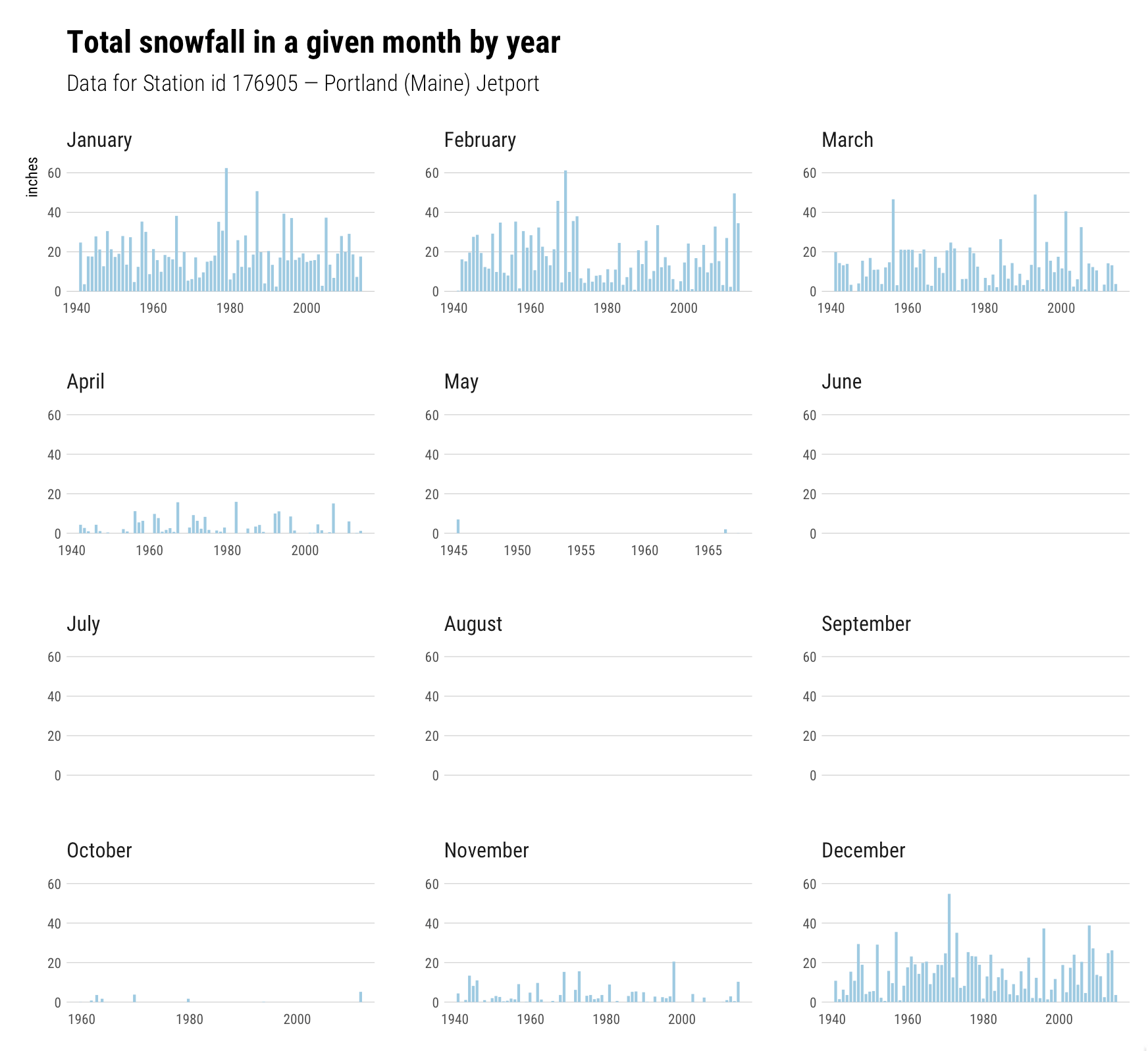# R⁶ — Snow Day Facets

Back in 2014 I blogged about first snowfall dates for a given U.S. state. It’s April 1, 2017 and we’re slated to get 12-18″ of snow up here in Maine and @mrshrbrmstr asked how often this — snow in May — has occurred near us.

As with all of these “R⁶ posts, expository is minimal and the focus is generally to demonstrate one small concept.

What I’ve done here (first) is make a full `tidyverse` update to the `snowfirst` code posted in the aforementioned blog post. You’ll need to clone that repo if you’re trying to work verbatim from the code below (otherwise just change file path code):

``````library(rprojroot)
library(stringi)
library(hrbrthemes)
library(tidyverse)

pre <- find_rstudio_root_file()

# Get and read in Maine precip ------------------------------------------------------

URL <- "http://cdiac.ornl.gov/ftp/ushcn_daily/state17_ME.txt.gz"
fil <- file.path(pre, "data", basename(URL))

col_positions = fwf_widths(c(6, 4, 2, 4, rep(c(5, 1, 1, 1), 31)),
col_names = c("coop_id", "year", "month", "element",
flatten_chr(map(1:31, ~paste("r_", c("v", "fm", "fq", "fs"),
.x, sep=""))))),
col_types = paste0("ciic", paste0(rep("iccc", 31), collapse=""), collapse=""),
na = c("", "NA", "-", "-9999")) %>%
gather(day, value, starts_with("r_v")) %>%
select(-starts_with("r_")) %>%
mutate(day = as.numeric(stri_replace_first_fixed(day, "r_v", ""))) %>%
mutate(date = sprintf("%s-%02d-%02d", year, month, day)) -> daily_wx

URL <- "http://cdiac.ornl.gov/ftp/ushcn_daily/ushcn-stations.txt"
fil <- file.path(pre, "data", basename(URL))

col_positions = fwf_widths(c(6, 9, 10, 7, 3, 31, 7, 7, 7, 3),
col_names = c("coop_id", "latitude", "longitude",
"elevation", "state", "name",
"component_1", "component_2",
"component_3", "utc_offset")),
col_types = "cdddcccccc") -> stations

closestStation <- function(stations, lat, lon, restrict_to = NULL) {
if (!is.null(restrict_to)) stations <- filter(stations, state == restrict_to)
index <- which.min(sqrt((stations\$latitude-lat)^2 +
(stations\$longitude-lon)^2))
stations[index,]
}

# compute total snow amounts per month ----------------------------------------------

(near_me <- closestStation(stations, 43.2672, -70.8617, restrict_to="ME"))``````

Now that we have the data, the short lesson here is just exposing the fact that you can get blank facets for free with ggplot2. I’m pointing this out as many folks seem to not like reading R documentation or miss things in said documentation (in fact, I had to be instructed today by @thomasp85 about a `ggplot2` theme element setting that I didn’t know about and should have since I do try to keep up).

``````filter(daily_wx, coop_id == near_me\$coop_id, element=="SNOW", value>0) %>%
count(year, month, wt=value) %>%
ungroup() %>%
mutate(
n = n / 10, # readings are in 10ths of inches
date = as.Date(sprintf("%s-%02d-01", year, month)),
month_name = lubridate::month(date, TRUE, FALSE)
) %>%
ggplot(aes(x=date, y=n)) +
geom_segment(aes(xend=date, yend=0), size=0.75, color="#9ecae1") +
scale_y_continuous(limits=c(0, 65)) +
facet_wrap(~month_name, ncol=3, drop=FALSE, scales="free") +
labs(x=NULL, y="inches", title="Total snowfall in a given month by year",
subtitle="Data for Station id 176905 — Portland (Maine) Jetport") +
theme_ipsum_rc(grid="Y", axis_text_size=8)``````Without `ggplot2` helping us out we would have had to do some work to have those no-value facets to show up. I also like how there are no x-axis labels since there’s no data. `ggplot2::facet_wrap()` has many, very granular options for customizing the appearance of facets:

``````facet_wrap(facets, nrow = NULL, ncol = NULL, scales = "fixed",
shrink = TRUE, labeller = "label_value", as.table = TRUE,
switch = NULL, drop = TRUE, dir = "h", strip.position = "top")``````

If you haven’t played with them, you can use this example to try them out.

### Fin

Even though that visualization gets the message across, I kinda like this view a bit better:

``````filter(daily_wx, coop_id == near_me\$coop_id, element=="SNOW", value>0) %>%
count(year, month, wt=value) %>%
ungroup() %>%
mutate(n = n / 10) %>%
complete(year, month=1:12) %>%
mutate(
date = as.Date(sprintf("%s-%02d-01", year, month)),
month_name = factor(lubridate::month(date, TRUE, FALSE), levels=rev(month.name))
) %>%
ggplot(aes(year, month_name)) +
geom_tile(aes(fill=n), color="#b2b2b2", size=0.15) +
scale_x_continuous(expand=c(0,0.15), position="top") +
viridis::scale_fill_viridis(name = "Total inches", na.value="white") +
labs(x=NULL, y=NULL, title="Total snowfall in a given month by year",
subtitle="Data for Station id 176905 — Portland (Maine) Jetport") +
theme_ipsum_rc(grid="", axis_text_size = 10) +
guides(fill=guide_colourbar(label.position = "top", direction = "horizontal", title.vjust = 0)) +
theme(legend.title = element_text(size=10)) +
theme(legend.key.height = unit(0.5, "lines")) +
theme(legend.position = c(0.9, 1.25))``````The precision is lacking in the heatmap view, but you get a quick impression of when it has/hasn’t snowed. Plus you get to use `viridis` ?

All the updated code in in the `snowfirst` repo.

Crank you your own, small code snippets or ideas to the R community. R⁶ is an open tag and perhaps we can band together to make a distributed cadre of helpful, digestible posts the R community can benefit from.Amazon Author Page

## 1 Comment →R⁶ — Snow Day Facets

1. Pingback: R⁴ — Snow Day Facets – Cyber Security

This site uses Akismet to reduce spam. Learn how your comment data is processed.